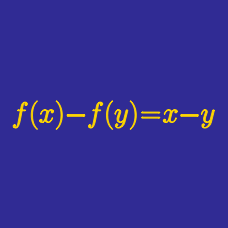Algebra

# Functional Equations: Level 4 Challenges

Let $F(x)$ be a non-decreasing function defined on $[0, 1 ]$ such that

$\begin{cases} 2 F \left ( \frac{x}{3} \right) = F(x), \\ F(x) + F(1-x) = 1 .\\ \end{cases}$

What is the value of $F ( \frac{1}{13} )$?

Find the number of functions $f : \mathbb{R} \rightarrow \mathbb{R}$ such that $f(x+y)=f(x)\cdot f(y)\cdot f(xy)$ for all $x,y$ in $\mathbb{R}$.

Note: $\mathbb{R}$ denotes the set of real numbers.

Let $f(x)$ be a polynomial such that

$f(f(x)) - x^{2} = xf(x).$

Find $f(-100)$.

##### This problem is posed by Zi Song Y.

$f(x)$ is an even function with codomain $\mathbb{R}.$ Its graph is symmetric about the line $x=1$, and $f(x_{1}+x_{2})=f(x_{1})\cdot f(x_{2})$ for any $x_{1},x_{2}\in [0,\frac{1}{2}]$, and $f(1)> 0.$

Let $a_{n}=f(2n+\frac{1}{2n})$. Find the value of $\displaystyle\lim_{n\rightarrow \infty }(\ln\: a_{n})$.

Find the number of functions such that $f:\mathbb{R}\rightarrow\mathbb{R}$ satisfying $f(x^{2} + yf(z))=xf(x) + zf(y)$ for all $x,y,z\in \mathbb{R}$.

×